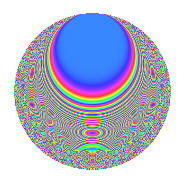# Properties

 Label 630.2.biLevel 630 Weight 2 Character orbit bi Rep. character $$\chi_{630}(479,\cdot)$$ Character field $$\Q(\zeta_{6})$$ Dimension 96 Newform subspaces 2 Sturm bound 288 Trace bound 2

# Related objects

## Defining parameters

 Level: $$N$$ = $$630 = 2 \cdot 3^{2} \cdot 5 \cdot 7$$ Weight: $$k$$ = $$2$$ Character orbit: $$[\chi]$$ = 630.bi (of order $$6$$ and degree $$2$$) Character conductor: $$\operatorname{cond}(\chi)$$ = $$315$$ Character field: $$\Q(\zeta_{6})$$ Newform subspaces: $$2$$ Sturm bound: $$288$$ Trace bound: $$2$$ Distinguishing $$T_p$$: $$13$$

## Dimensions

The following table gives the dimensions of various subspaces of $$M_{2}(630, [\chi])$$.

Total New Old
Modular forms 304 96 208
Cusp forms 272 96 176
Eisenstein series 32 0 32

## Trace form

 $$96q + 96q^{4} + O(q^{10})$$ $$96q + 96q^{4} + 12q^{11} + 6q^{14} + 2q^{15} + 96q^{16} + 16q^{21} - 6q^{29} - 10q^{30} - 30q^{35} - 36q^{39} - 6q^{41} + 12q^{44} - 24q^{45} + 6q^{46} + 6q^{49} - 36q^{50} + 20q^{51} + 6q^{56} + 2q^{60} + 96q^{64} - 84q^{69} + 6q^{70} + 36q^{75} + 16q^{81} + 16q^{84} - 66q^{89} - 54q^{90} - 52q^{99} + O(q^{100})$$

## Decomposition of $$S_{2}^{\mathrm{new}}(630, [\chi])$$ into newform subspaces

Label Dim. $$A$$ Field CM Traces $q$-expansion
$$a_2$$ $$a_3$$ $$a_5$$ $$a_7$$
630.2.bi.a $$48$$ $$5.031$$ None $$-48$$ $$0$$ $$0$$ $$-3$$
630.2.bi.b $$48$$ $$5.031$$ None $$48$$ $$0$$ $$0$$ $$3$$

## Decomposition of $$S_{2}^{\mathrm{old}}(630, [\chi])$$ into lower level spaces

$$S_{2}^{\mathrm{old}}(630, [\chi]) \cong$$ $$S_{2}^{\mathrm{new}}(315, [\chi])$$$$^{\oplus 2}$$

## Hecke Characteristic Polynomials

There are no characteristic polynomials of Hecke operators in the database